## Recommended Posts

Hello,

Currently I'm trying to work on a project that should allow me to find the biggest matches quite easily by analyzing photos of some kind of "ballot paper".

Here is a picture of a possible ballot.

At the moment I'm looking for a way to get the position of the red dots and the black crosses. I'm trying to use PixelSearch, but would be very grateful for your tips.

The final goal would be to convert every single "ballot paper" (on the photo you can see 6 next to each other) into an array with the respective selections (\$array = [1, 9, 89]).

Edited by tz45

##### Share on other sites
19 hours ago, tz45 said:

(\$array = [1, 9, 89]).    -->   (\$array = [1, 9, 87]).   ???

UDF: _SingleScript()                                        If you like my post, just click the like buttonhere -->

##### Share on other sites

As I'm new to this forum I can't edit my post yet##### Share on other sites

I have to admit that I'm quite astonished that I didn't even get a single hint or tip hereMeanwhile, I solved it myself by using FastFind. There are now a total of 12 red dots on the ballot paper helping the algorithm to find the corners and exact coordinates of the black dots using a little analytical geometry and arithmetic.

As o utput I get an array with all results, which I can then use further.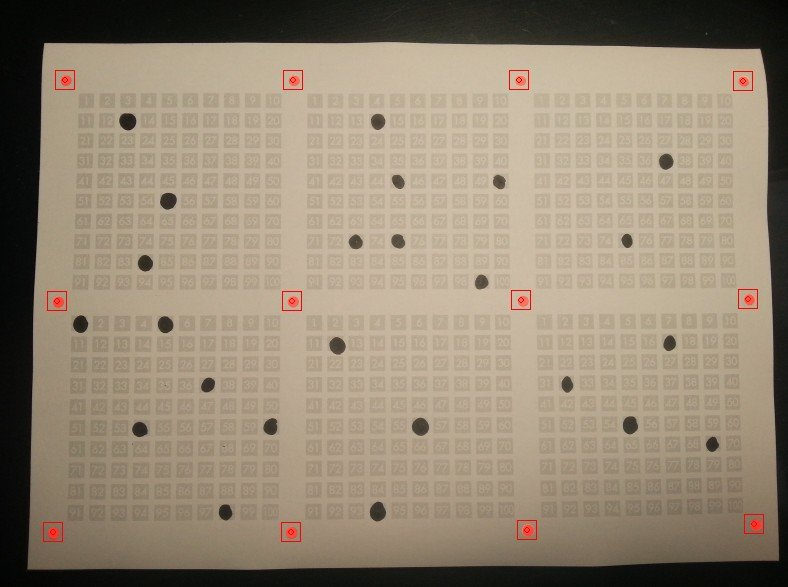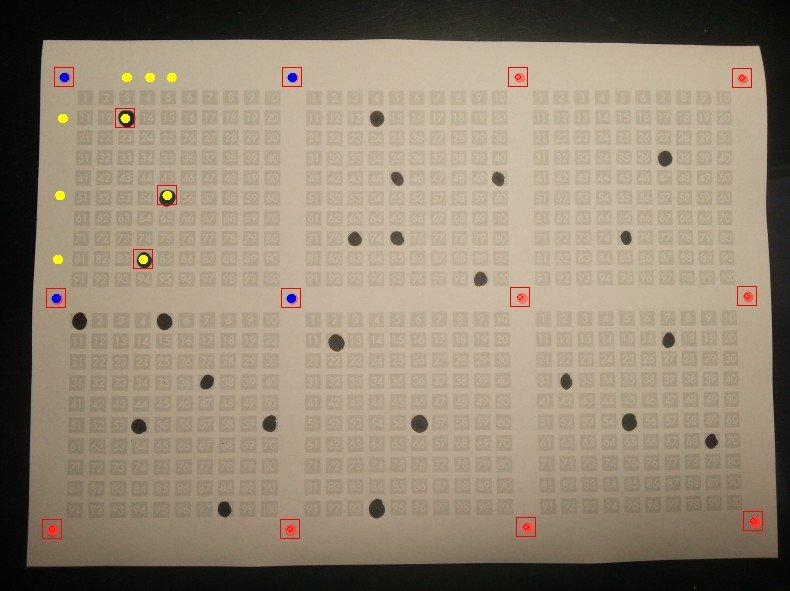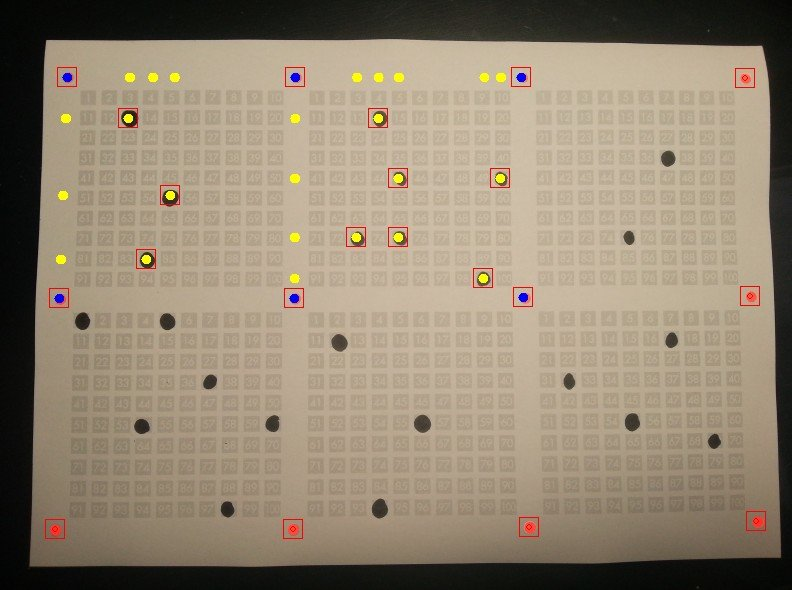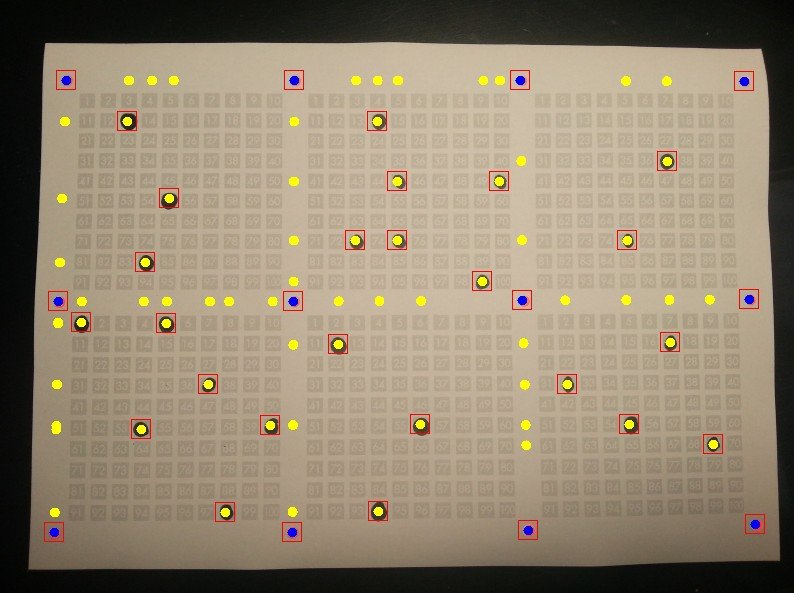##### Share on other sites

EDITED

Content of

`ExtractDots.au3`
```#include <Array.au3>
#include <CustomMsgBox.au3>
#include <FastFind.au3>
#include <File.au3>
#include <GuiConstants.au3>
#include <GUIConstantsEx.au3>
#Include <GDIPlus.au3>
#Include <WinAPI.au3>

Global \$hGui, \$hgraphic
Global \$w = 800
Global \$h = 600
Global \$pos
Global \$grid
Global \$dotValues
Global \$colorThreshold = [20000, 25000]

Func ExtractDots(\$imgFile)
Do
Global \$dotValues

\$tmpFile = PrepareImg(\$imgFile)
Gui(\$tmpFile)

for \$i = 0 to 11
FindDot(1, \$i)
Next

for \$i = 1 to 6
SetBallot(\$i)

Local \$dots
While 1
\$result = FindDot()

if \$result == false then _
ExitLoop

_ArrayAdd(\$dots, \$result & "|" & \$result)
WEnd

DotsToValue(\$i, \$dots)
Next

Until \$isDone

_GDIPlus_Shutdown()
GUIDelete()
DirRemove("tmp", 1)

return \$dotValues
EndFunc

\$colorReturn = xMsgBox(16+0x200, _
"Black <", "Done", "> Red", _
Default, \$pos + \$pos + 5)

if \$colorReturn = 7 then _
return true

\$colorIndex = (\$colorReturn == 6) _
? 0 _
: 1

\$fuzzReturn = xMsgBox(16+0x200, _
"Less <", "Done", "> More", _
Default, \$pos + \$pos + 5)

\$factor = (\$fuzzReturn == 6) _
? -1 _
: 1
\$colorThreshold[\$colorIndex] += \$factor * 2500

return false
EndFunc

Func PrepareImg(\$imgFile)
Local \$sDrive = "", \$sDir = "", \$sFileName = "", \$sExtension = ""
Local \$aPathSplit = _PathSplit(\$imgFile, \$sDrive, \$sDir, \$sFileName, \$sExtension)
\$tmpFile = @ScriptDir & "\tmp\" & \$sFileName & \$sExtension

Local \$magickCmds = [ _
"magick convert """ & \$imgFile & """ -fuzz " & \$colorThreshold & " -fill red -opaque red """ & \$tmpFile & """", _
"magick convert """ & \$tmpFile & """ -fuzz " & \$colorThreshold &" -fill black -opaque black """ & \$tmpFile & """" ]

DirCreate("tmp")
for \$cmd in \$magickCmds
RunWait(\$cmd, @ScriptDir, @SW_HIDE)
Next

return \$tmpFile
EndFunc

Func Gui(\$tmpFile)
_GDIPlus_Startup()
Global \$hGui
\$hBitmap = _GDIPlus_BitmapCreateFromFile(\$tmpFile)

\$hGui = GUICreate("ExtractDots", \$w, \$h, -1, -1, \$WS_POPUP)
GUISetState()

\$pos = WinGetPos("ExtractDots")

\$hgraphic = _GDIPlus_GraphicsCreateFromHWND(\$hGui)
_GDIPlus_GraphicsDrawImageRect(\$hgraphic, \$hBitmap, 0, 0, \$w, \$h)
_GDIPlus_BitmapDispose(\$hBitmap)

FFSetWnd(\$hGui)
FFSnapShot()
EndFunc

Func DrawPoint(\$coords, \$colorIndex = 0)
Local \$colors = [ _
0xFF0ca2f0, _
0xFFFFFF00, _
0xFFFF0000, _
0xFFFF00cc, _
0xFF66FF00  _
]

\$colorIndex = Mod(\$colorIndex, UBound(\$colors))
\$color = \$colors[\$colorIndex]

\$hBrush = _GDIPlus_BrushCreateSolid(\$color)
_GDIPlus_GraphicsFillEllipse(\$hgraphic, _
\$coords - 5, _
\$coords - 5, _
10, 10, \$hBrush)
EndFunc

Func DotsToValue(\$ballotIndex, \$dots)
\$corners = GetCorners(\$ballotIndex)

\$first = SingleCorner(\$corners, 0)
\$second = SingleCorner(\$corners, 1)
\$third = SingleCorner(\$corners, 2)
\$fourth = SingleCorner(\$corners, 3)
DrawPoint(\$first)
DrawPoint(\$second)
DrawPoint(\$third)
DrawPoint(\$fourth)

Local \$values
for \$i = 0 to UBound(\$dots) - 1
Local \$dot
_ArrayAdd(\$dot, \$dots[\$i] & "|" & \$dots[\$i])
DrawPoint(\$dot, 1)

\$xVanishingPoint = GetPOI(\$first, \$third, \$second, \$fourth)
if Not \$xVanishingPoint then
\$vector = VectorCalc(\$first, '-', \$third)
\$xVanishingPoint = VectorCalc(\$dot, '+', \$vector)
EndIf

\$yVanishingPoint = GetPOI(\$first, \$second, \$third, \$fourth)
if Not \$yVanishingPoint then
\$vector = VectorCalc(\$first, '-', \$second)
\$yVanishingPoint = VectorCalc(\$dot, '+', \$vector)
EndIf

\$xPOI = GetPOI(\$first, \$second, \$dot, \$xVanishingPoint)
\$yPOI = GetPOI(\$first, \$third, \$dot, \$yVanishingPoint)

;DrawPoint(\$xPOI, 4)
;DrawPoint(\$yPOI, 4)

\$xVector = VectorCalc(\$xPOI, '-', \$first)
\$yVector = VectorCalc(\$yPOI, '-', \$first)

\$xLength = VectorCalc(\$xVector, '|')
\$top = VectorCalc(\$second, '-', \$first)
\$topLength = VectorCalc(\$top, '|')
\$xPercentage = \$xLength / \$topLength
\$xDigit = Round(\$xPercentage * 11)

\$yLength = VectorCalc(\$yVector, '|')
\$left = VectorCalc(\$third, '-', \$first)
\$leftLength = VectorCalc(\$left, '|')
\$yPercentage = \$yLength / \$leftLength
\$yDigit = Round(\$yPercentage * 11)

\$value = (\$yDigit - 1)*10 + \$xDigit

Next

_ArraySort(\$values)
EndFunc

Func GetPOI(\$startPoint1, \$endPoint1, \$startPoint2, \$endPoint2)
\$a = \$startPoint1
\$b = \$startPoint1
\$vector1 = VectorCalc(\$endPoint1, '-', \$startPoint1)
\$c = \$vector1
\$d = \$vector1

\$e = \$startPoint2
\$f = \$startPoint2
\$vector2 = VectorCalc(\$endPoint2, '-', \$startPoint2)
\$m = \$vector2
\$n = \$vector2

if \$d*\$m - \$c*\$n == 0 then _
return false

\$s = (-\$n*(\$e - \$a) + \$m*(\$f - \$b)) / (\$d*\$m - \$c*\$n)
\$x = \$a + \$s*\$c
\$y = \$b + \$s*\$d

Local \$result = [\$x, \$y]
return \$result
EndFunc

Func SingleCorner(\$array, \$index)
Local \$result

for \$i = 0 to 1
\$result[\$i] = \$array[\$index][\$i]
Next

return \$result
EndFunc

Func SetBallot(\$index)
\$corners = GetCorners(\$index)

SetArea( _
\$corners, \$corners, _
\$corners, \$corners)
EndFunc

Func GetCorners(\$index)
Local \$corners

\$missedSquare = Floor((\$index - 1) / 3)
\$corners = \$index - 1 + \$missedSquare
\$corners = \$corners + 1
\$corners = \$index + 3 + \$missedSquare
\$corners = \$corners + 1

Local \$result
for \$i = 0 to 3
\$entryIndex = \$corners[\$i]
\$cornerCoordinates = GetGridEntry(\$entryIndex)

for \$j = 0 to 1
\$result[\$i][\$j] = \$cornerCoordinates[\$j]
Next
Next

return \$result
EndFunc

Func SetGridEntry(\$array, \$index)
for \$i = 0 to 1
\$grid[\$index][\$i] = \$array[\$i]
Next
EndFunc

Func GetGridEntry(\$index)
Local \$array

For \$i = 0 to 1
\$array[\$i] = \$grid[\$index][\$i]
Next

return \$array
EndFunc

Func VectorCalc(\$par1, \$operation, \$par2 = 0)
switch \$operation
case '+'
Local \$result
for \$i = 0 to 1
\$result[\$i] = \$par1[\$i] + \$par2[\$i]
Next
case '-'
Local \$result
for \$i = 0 to 1
\$result[\$i] = \$par1[\$i] - \$par2[\$i]
Next
case '*'
Local \$result
for \$i = 0 to 1
\$result[\$i] = \$par1 * \$par2[\$i]
Next
case '|'
Local \$result = Sqrt(\$par1^2 + \$par1^2)
EndSwitch

return \$result
EndFunc

Func SetArea(\$a = -1, \$b = -1, \$c = -1, \$d = -1)
FFResetExcludedAreas()

if \$a + \$b + \$c + \$c == -4 then _
return

;top
0, _
0, _
\$w, _
\$b)

;left
0, _
\$b, _
\$a, _
\$d)

;right
\$c, _
\$b, _
\$w, _
\$d)

;bottom
0, _
\$d, _
\$w, _
\$h)
EndFunc

Func FindDot(\$isRed = 0, \$gridIndex = -1)
? 250 _
: 60
local \$result
Local \$color = (\$isRed) _
? 0xFFFF0000 _
: 0xFF000000

if \$isRed then
\$horizontalFactor = Mod(\$gridIndex, 4)
\$firstX = \$horizontalFactor * \$w/4
\$secondX = \$firstX + \$w/4

\$verticalFactor = Floor(\$gridIndex/4)
\$firstY = \$verticalFactor * \$h/3
\$secondY = \$firstY + \$h/3

SetArea(\$firstX, \$firstY, \$secondX, \$secondY)
EndIf

do
\$result = FFNearestSpot(20, 50, 0, 0, \$color, \$shadeVariation, 0)

if (Not IsArray(\$result)) Then _

if Not IsArray(\$result) then _
return false

if \$isRed then
SetGridEntry(\$result, \$gridIndex)
Else
\$result - 20, _
\$result - 20, _
\$result + 20, _
\$result + 20)
EndIf

return _ArrayExtract(\$result, 0, 1)
EndFunc```

Can be called from another project like this:

```#include <ExtractDots.au3>

Local \$files = [ _
"example3.jpg", _
"example5.jpg", _
"example9.jpg" ]

for \$file in \$files
\$values = ExtractDots(\$file)
Next

Depending on the brightness, saturation, and illumination of the image, individual points may not or too many may be detected.

Therefore, using ImageMagick (must be installed), each image is edited to highlight red and black.

Each image is followed by a query that allows you to adjust the two colors.

Here are some files to try out.

ExtractDots.zip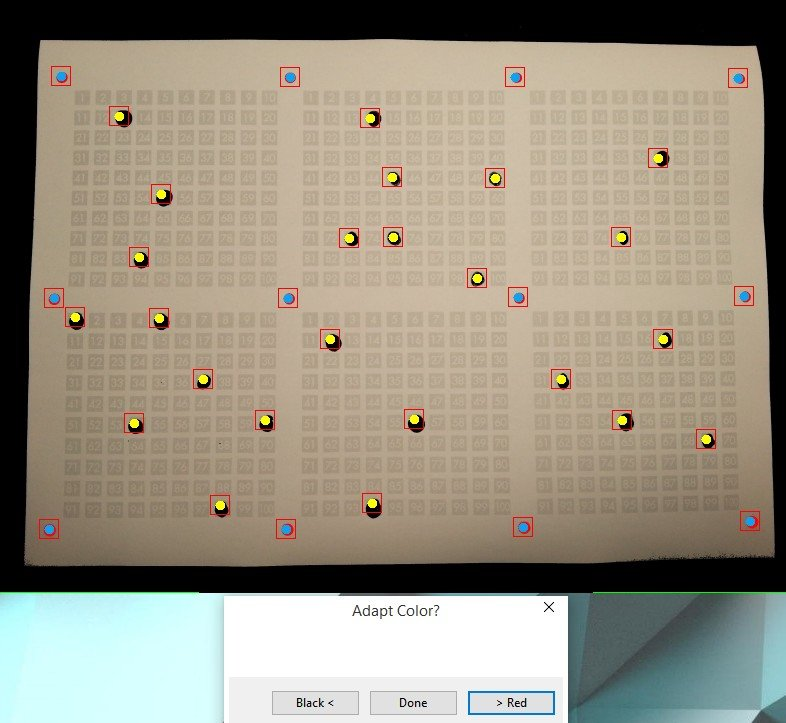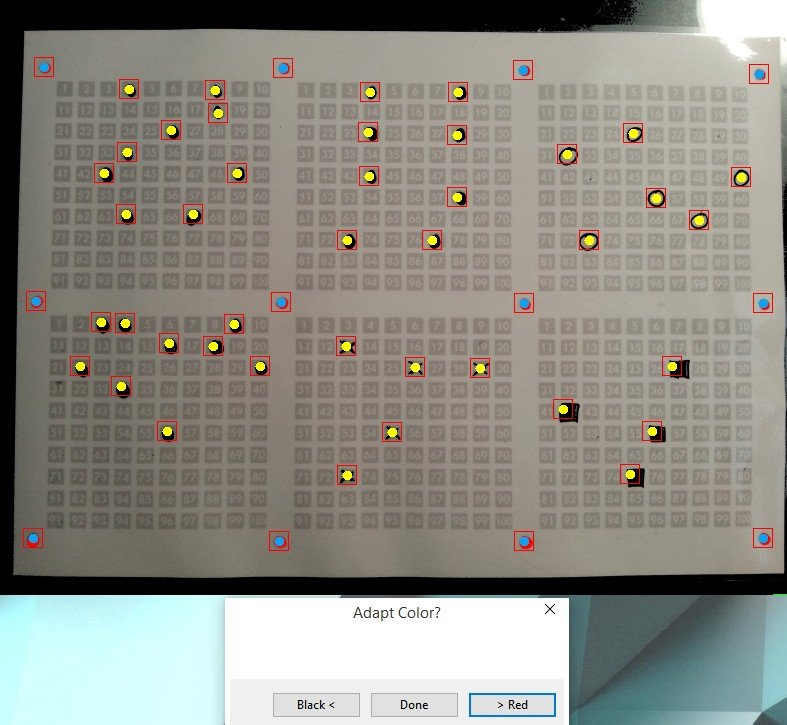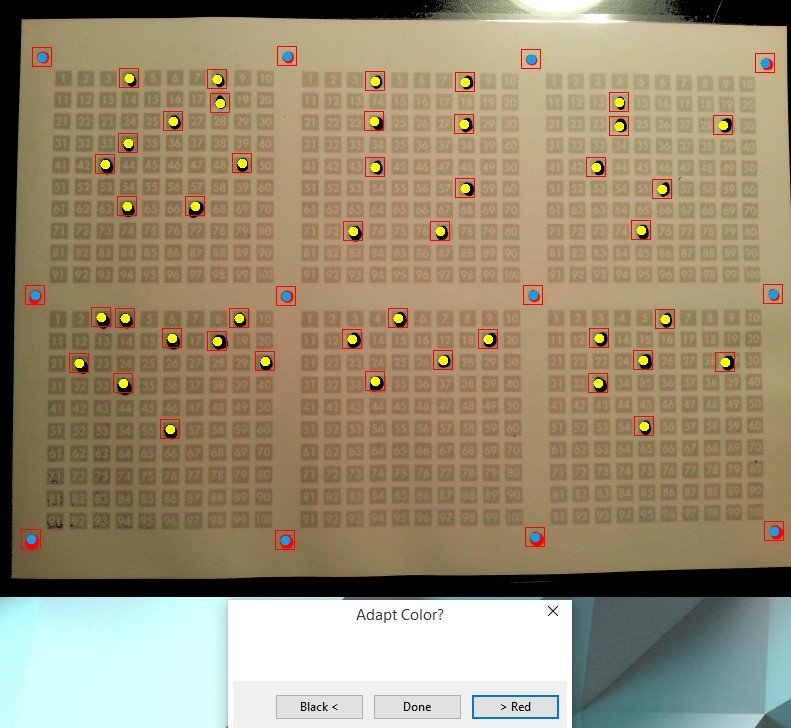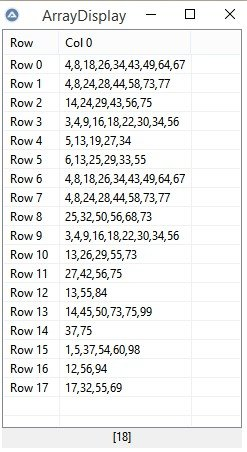Edited by tz45

## Create an account

Register a new account

×

• Wiki

• Back

• #### Beta

• Git
• FAQ
• Our Picks
×
• Create New...